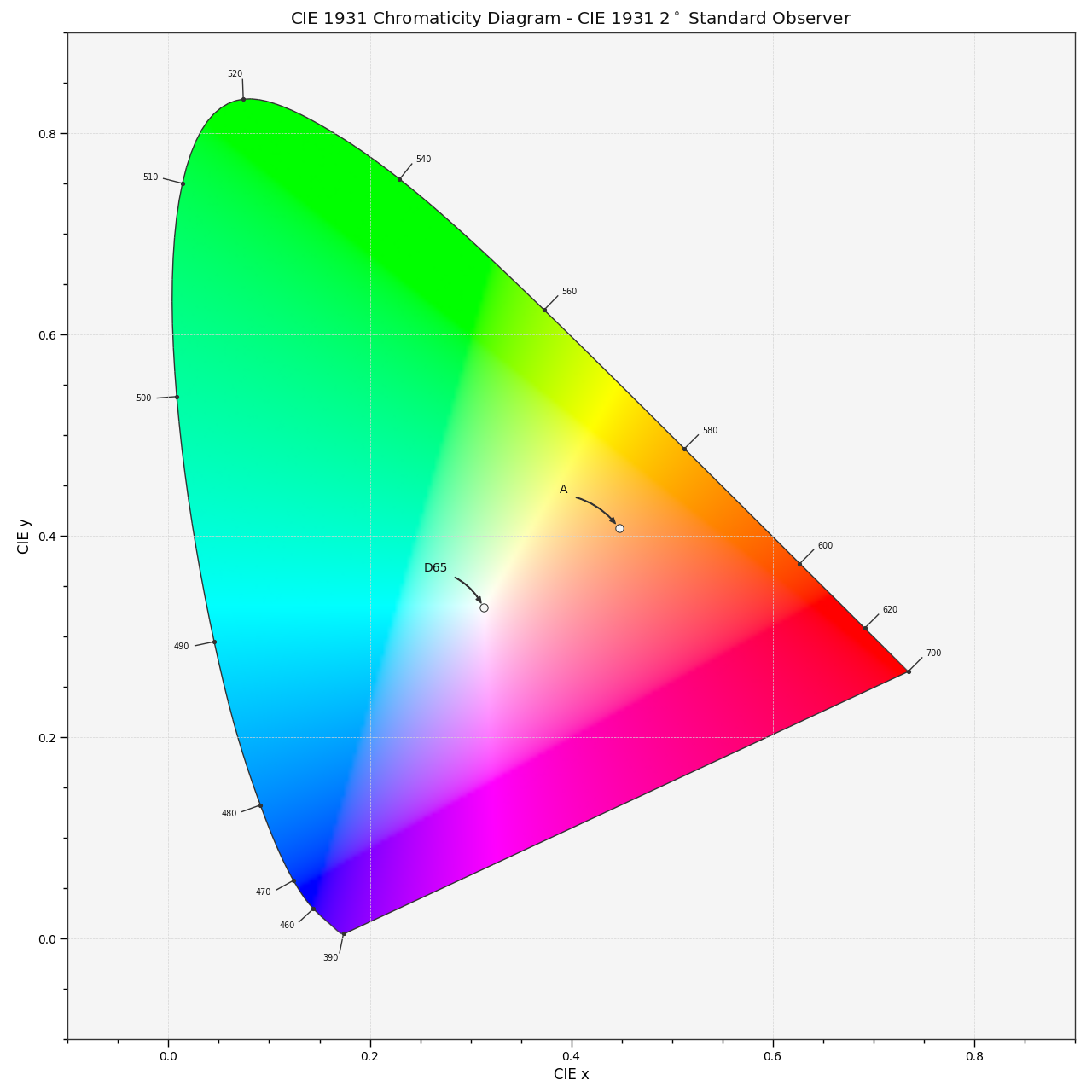# colour.plotting.plot_sds_in_chromaticity_diagram_CIE1931#

colour.plotting.plot_sds_in_chromaticity_diagram_CIE1931(sds: , cmfs: = 'CIE 1931 2 Degree Standard Observer', chromaticity_diagram_callable_CIE1931: Callable = plot_chromaticity_diagram_CIE1931, annotate_kwargs: dict | List[dict] | None = None, plot_kwargs: dict | List[dict] | None = None, **kwargs: Any) [source]#

Plot given spectral distribution chromaticity coordinates into the CIE 1931 Chromaticity Diagram.

Parameters:
Returns:

Current figure and axes.

Return type:

`tuple`

Examples

```>>> A = SDS_ILLUMINANTS["A"]
>>> D65 = SDS_ILLUMINANTS["D65"]
>>> plot_sds_in_chromaticity_diagram_CIE1931([A, D65])
...
(<Figure size ... with 1 Axes>, <...Axes...>)
```